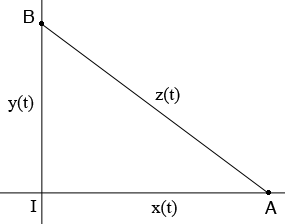SEARCH HOMEMath Central Quandaries & QueriesQuestion from Nuraini, a student: Two straight roads intersect at the right angles. Lorry A, moving on one of the roads, Approaches the intersection at 50mi/h and lorry B, moving on the other roads, approaches the intersection at 20mi/h. At what rate is the distance between the lorry changing when A is 0.4 mile from the intersection and B is 0.3 mile from the intersection?Hi Nuraini,

Here is my diagram of the situation you describe at an arbitrary time $t$ hours.$A$ and $B$ show the position of the lorries and $I$ is the intersection. $x(t)$ is the distance from lorry $A$ to the intersection, $y(t)$ is the distance from lorry $B$ to the intersection and $z(t)$ is the distance between the lorries, all in miles. Since triangle $BIA$ is a right triangle Pythagoras Theorem gives

$z^2(t) = x^2(t) + y^2(t).$

Differentiate both sided with respect to $t$ and you will obtain an equation relating all three distanced and their derivatives at an arbitrary time $t$ hours.

Now focus on the time when lorry $A$ is 0.4 miles from the intersection and lorry B is 0.3 miles from the intersection. At this time you know the values of all the variables in the derivative equation except $z^\prime(t).$ Solve for $z^\prime(t).$

PennyMath Central is supported by the University of Regina and the Imperial Oil Foundation.